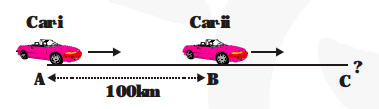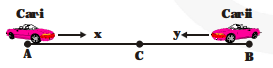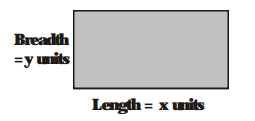# Form the pair of linear equations in the following problems and find their solutions (if they exist) by any algebraic method

Question.

Form the pair of linear equations in the following problems and find their solutions (if they exist) by any algebraic method

(i) A part of monthly hostel charges is fixed and the remaining depends on the number of days one has taken food in the mess. When a student A takes food for 20 days she has to pay Rs. 1000 as hostel charges whereas a student B, who takes food for 26 days, pays Rs. 1180 as hostel charges. Find the fixed charges and the cost of food per day.

(ii) A fraction becomes $\frac{\mathbf{1}}{\mathbf{3}}$ when 1 is subtracted from the numerator and it becomes $\frac{\mathbf{1}}{\mathbf{4}}$ when

8 is added to its denominator. Find the fraction.

(iii) Yash scored 40 marks in a test, getting 3 marks for each right answer and losing 1 mark for each wrong answer. Had 4 marks been awarded for each correct answer and 2 marks been deducted for each incorrect answer, then Yash would have scored 50 marks. How many questions were there in the test?

(iv) Places A and B are 100 km apart on a highway. One car starts from A and another from B at the same time. If the cars travel in the same direction at different speeds, they meet in 5 hours. If they travel towards each other, they meet in 1 hour. What are the speeds of the two cars?

(v) The area of a rectangle gets reduced by 9 square units if its length is reduced by 5 units and breadth is increased by 3 units. If we increase the length by 3 units and the breadth by 2 units, the area increases by 67 square units. Find the dimensions of the rectangle.

Solution:

(i) Let the fixed charge be x

and charges of food for 1 day be y.

So, x + 20y = 1000 ..........(i)

x + 26y = 1180 ..........(ii)

Subtracting (i) from (ii), we get

6y = 180 ; y = 30

Substituting y in (i), we get

x + 20 × 30 = 1000

x = 1000 – 600

x = 400

So, fixed charge = Rs.400 and charges of food

for 1 day = Rs.30

(ii) Let the fraction be $\frac{\mathbf{x}}{\mathbf{y}}$

Then, $\frac{\mathbf{x}-\mathbf{1}}{\mathbf{y}}=\frac{\mathbf{1}}{\mathbf{3}}$ ...(i)

$\frac{x}{y+8}=\frac{1}{4}$ ....(ii)

From (i) and (ii), we get

3x – 3 = y or 3x – y = 3 ......(iii)

4x = y + 8 or 4x – y = 8 ......(iv)

Subtracting (iii) from (iv), we get

4x – y – 3x + y = 5

x = 5

Substituting x in (iii), we get

3 × 5 – y = 3

y = 12

So the required fraction is $\frac{\mathbf{5}}{\mathbf{1 2}}$

(iii) Number of right answers = x. Number

Then, 3x – y = 40 ...(i)

4x – 2y = 50 ...(ii)

Multiplying (i) by 2, we get

6x – 2y = 80 ...(iii)

Subtracting (ii) from (iii), we get

2x = 30

= x = 15

Substituting x = 15, in (i), we get

45 – y = 40

= y = 5

Total questions = x + y = 15 + 5 = 20

(iv) Speed of car i = x km/hr

Speed of car ii = y km/hr

First case :Two cars meet at C after 5 hrs.

AC – BC = AB

= 5x – 5y = 100 ...(i)

Second case :Two cars meet at C after one hour

x + y = 100 ...(ii)

Multiplying (ii) by 5, we get

5x + 5y = 500 ...(iii)

Adding (i) and (iii), we get

10x = 600

= x = 60 km/hr

Substituting x = 60 km/hr in (ii), we get

y = 40 km/hr

Speed of car (i) = 60 km/hr

Speed of car (ii) 40 km/hr

(v) In first case, area is reduced by 9 square unitsWhen length = x – 5 units

and breadth = y + 3 units

= xy – (x – 5) × (y + 3) = 9 ...(i)

In second case area increases by 67 sq. units when length = x + 3 and breadth = y + 2.

= (x + 3) × (y + 2) – xy = 67 ...(ii)

Solving both equations, we get

xy – xy – 3x + 5y + 15 = 9

3x – 5y = 6 ...(iii)

xy + 2x + 3y + 6 – xy = 67

2x + 3y = 61 ...(iv)

Multiplying (iii) by 3 and (iv) by 5, we get

9x – 15y = 18

10x + 15y = 305